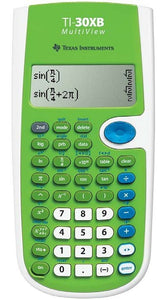# TI-30XB MultiView™

Regular price
HKD150.00
Sale price
HKD150.00
Regular price
Sold out
Unit price
per

### TI-30XB MultiView™ Scientific Calculator

Designed with unique features that allow you to enter more than one calculation, compare results and explore patterns, all on the same screen, the TI-30XB MultiView™ is ideal for the early secondary maths and science classroom.

Key features

• Four-line display
• One- and two-variable statistics
• MathPrint™ feature
• Fraction/decimal conversion
• Fraction math
• Edit, cut and paste entries
• Battery powered

View more calculations at a time
Four-line display allows you to enter more than one calculation, compare results and explore patterns, all on the same screen.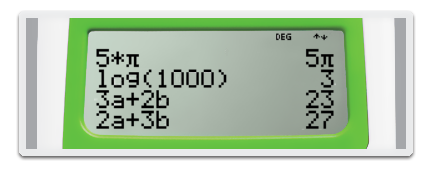MathPrint™ feature
Use the MathPrint™ feature to display expressions, symbols, and fractions just as they appear in textbooks.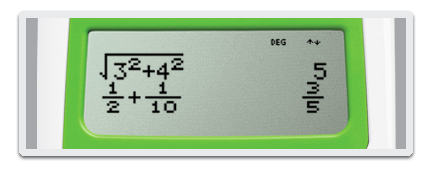Scientific notation output
View scientific notation with the proper superscripted exponents and see the output in scientific notation.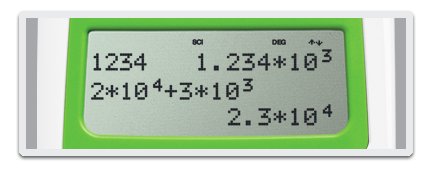Explore (x,y) table of values
Students can easily explore an (x,y) table of values for a give n function, automatically or by entering specific x values.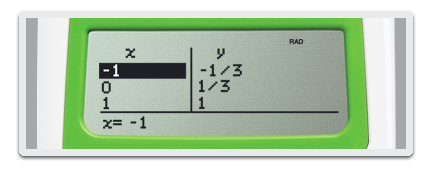Built-in functionality

• Review and edit previous entries
• Fraction/decimal conversions
• Random number and random integer generator
• Negation key
• Menu settings
• Change improper fractions to mixed numbers
• Automatic simplification of fractions
• One constant
• Combinations and permutations
• Trigonometry
• Hyperbolics
• Logs and antilogs
• Convert angles from degrees to radians to grads
• %, x², ¹/x, yˆx, π, x!
• Fixed decimal capability
• One- and two- variable statistics
• EOS: (Equation Operating System)
• Up to eight pending operations
• Up to 23 levels of parentheses
• Error recovery capability
• Five memory variables
• Scientific and engineering notation